# Texas Go Math Grade 8 Lesson 13.2 Answer Key Algebraic Representations of Dilations

Refer to our Texas Go Math Grade 8 Answer Key Pdf to score good marks in the exams. Test yourself by practicing the problems from Texas Go Math Grade 8 Lesson 13.2 Answer Key Algebraic Representations of Dilations.

## Texas Go Math Grade 8 Lesson 13.2 Answer Key Algebraic Representations of Dilations

Explore Activity 1

Graphing Enlargements

When a dilation in the coordinate plane has the origin as the center of dilation, you can find points on the dilated image by multiplying the x- and y-coordinates of the original figure by the scale factor. For scale factor k, the algebraic representation of the dilation is (x, y) → (kx, ky).
For enlargements, k > 1.

The figure shown on the grid is the preimage. The center of dilation is the origin.A. List the coordinates of the vertices of the preimage in the first column of the table.B. What is the scale factor for the dilation?
C. Apply the dilation to the preimage and write the coordinates of the vertices of the image in the second column of the table.
D. Sketch the image after the dilation on the coordinate grid.

Reflect

Question 1.
How does the dilation affect the length of line segments?
Dilation is a process of changing the size of an object while the object remains in the same Shape. Depending on the scale factor, dilation may increase or decrease the starting object.

Therefore, we can conclude that dilation affects the length of line segments by either increasing or decreasing their length.

Dilations on the Coordinate Plane Answer Key Math 8th Grade Question 2.
How does the dilation affect angle measures?
Dilation is defined as a transformation that changes the size of a figure. A dilation can produce a larger figure an enlargement a smaller figure or a reduction.

Since it changes the size of a figure, it has nothing to do with the changes when it comes to the angles of the image. The measures of the angles of dilated figures remain the same as in the original image.

Thus, the dilation has no effect on the measures of the angles of the image.

Explore Activity 2

Graphing Reductions

For scale factors between 0 and 1, the image is smaller than the preimage. This is called a reduction.

The arrow shown is the preimage. The center of dilation is the origin.
A. List the coordinates of the vertices of the preimage in the first column of the table.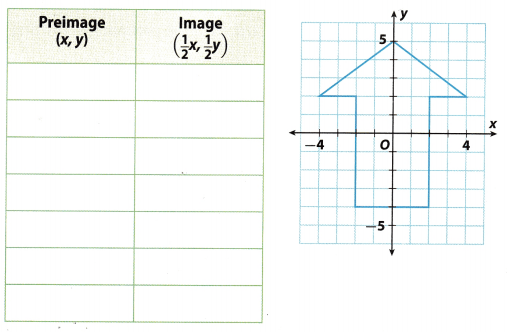B. What is the scale factor for the dilation?
C. Apply the dilation to the preimage and write the coordinates of the vertices of the image in the second column of the table.
D. Sketch the image after the dilation on the coordinate grid.

Reflect

Question 3.
How does the dilation affect the length of line segments?
A dilation can produce a larger figure (an enlargement) or a smaller figure (a reduction).

The dilation affects the length of line segments. If the dilation produces a larger figure the line segments of the dilated figure are longer than the original figure Also, if the dilation produces a smaller figure, the line segments of the dilated figure are smaller than the original figure.

Question 4.
How would a dilation with scale factor 1 affect the preimage?
A dilation can produce a larger figure (an enlargement) or a smaller figure (a reduction). The scale factor k describes how much the figure is enlarged or reduced. If the scale factor k is between 0 and 1 the image is a reduction. If the scale factor is greater than 1, the image is an enlargement.

By definition a dilation can only be enlarged or reduced with respect to the scale factor k between 0 and 1 or greater than 1, respectively, if the scale factor in the dilation is equal to 1, then the image would be the same as the preimage. There will be no change in their sizes and has no effect on the preimage.

Question 5.
Graph the image of △XYZ after a dilation with a scale factor of $$\frac{1}{3}$$ and the origin as its center. Then write an algebraic rule to describe the dilation.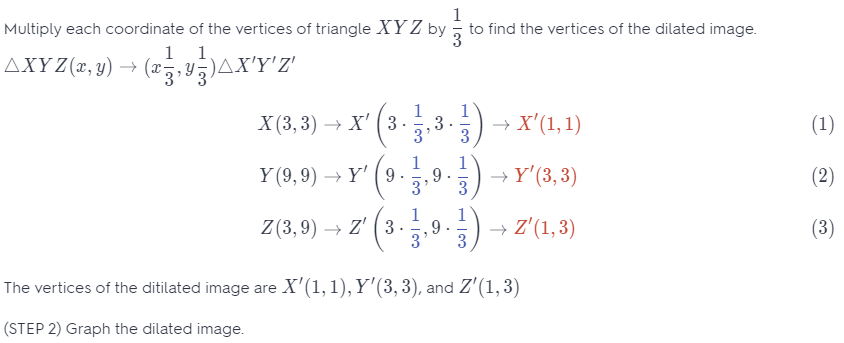The vertices of the dilated image are X'(1, 1), Y'(3, 3) and Z'(1, 3)

The grid shows a diamond-shaped preimage. Write the coordinates of the vertices of the preimage in the first column of the table. Then apply the dilation (x, y) → ($$\frac{3}{2}$$x, $$\frac{3}{2}$$y) and write the coordinates of the vertices of the image in the second column. Sketch the image of the figure after the dilation. (Explore Activities 1 and 2)Firstly, we will write the coordinates of the vertices of the preimage. To calculate the vertices of the image after dilation, we will use the following rule:
(x, y) ⇒ ($$\frac{3}{2}$$x, $$\frac{3}{2}$$y)
meaning that we will multiply every coordinate with $$\frac{3}{2}$$.

Filled table is shown in the picture below.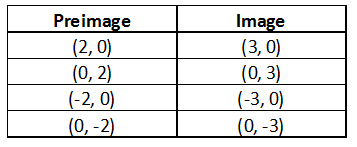After calculating the coordinates, we can graph every point of the image after dilation and connect them to get the wanted figure. The picture below shows the image of the figure before and after dilation.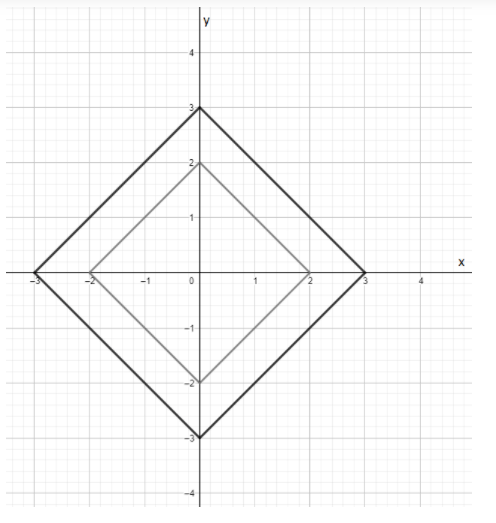Graph the image of each figure after a dilation with the origin as its center and the given scale factor. Then write an algebraic rule to describe the dilation. (Example 1)

Question 2.
The scale factor of 1.5Multiply each coordinate of the vertices of rectangle FGHI by 1, 5 to find the vertices of the dilated image.
Rectangle FGHI(x, y) → (1.5x, 1.5y)
F(2, 2) → F'(2 ∙ 1.5, 2 ∙ 1.5) → F'(3, 3) ……………….. (1)
G(5, 2) → G'(5 ∙ 1.5, 2 ∙ 1.5) → G'(7.5, 3) …………… (2)
I(2, 4) → I'(2 ∙ 1.5, 4 ∙ 1.5) → I'(3, 6) ………………… (3)
H(5, 4) → H'(5 ∙ 1.5, 4 ∙ 1.5) → H'(7.5, 6) …………… (4)
The vertices of the dilated image are F'(3.5, 3.5), G'(7.5, 3.5), H'(7.5, 6) and I'(, 9).

Graph the dilated image.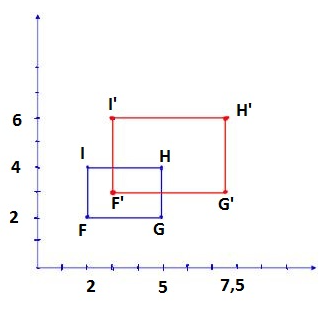Question 3.
scale factor of $$\frac{1}{3}$$Multiply each coordinate of the vertices of triangle ABC by $$\frac{1}{3}$$ to find the vertices of the dilated image.
△ABC(x,y) —* (x,y.)△A’B’C’
A(3, 3) → A'(3 ∙ $$\frac{1}{3}$$, 3 ∙ $$\frac{1}{3}$$) → A'(1, 1) ………….. (1)
B(9, 3) → B'(9 ∙ $$\frac{1}{3}$$, 3 ∙ $$\frac{1}{3}$$) → B'(3, 1) ………….. (2)
C(3, 9) → C'(3 ∙ $$\frac{1}{3}$$, 9 ∙ $$\frac{1}{3}$$) → C'(1, 3) …………… (3)
The vertices of the dilated image are A'(1, 1), B'(3, 1), and C'(1, 3)

Graph the dilated image.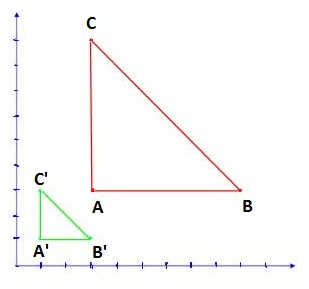Essential Question Check-In

A dilation of (x, y) → (kx, ky) when 0 < k < 1 has what effect on the figure? What is the effect on the figure when k > 1?
Explanation A:
When 0 < k < the dilation is a reduction of the original by the scale k. What k > 1 the dilation is an enlargement of the original by the scale k.

Explanation B:
When k is between 0 and 1, the dilation is a reduction by the scale factor k.
When k is greater than 1, the dilation is an enlargement by the scale factor k.

Question 5.
The blue square is the preimage. Write two algebraic representations, one for the dilation to the green square and one for the dilation to the purple square.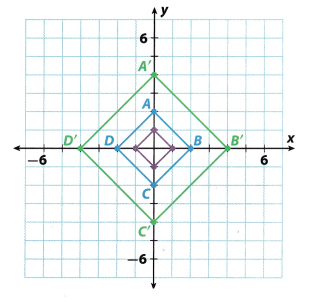The blue square ABCD is the preimage.
The coordinates of the vertices of the original image are multiplied by 2 for the green square.
(x, y) → (2x, 2y)
The coordinates of the vertices of the original image are multiplied by $$\frac{1}{2}$$ for the purple square.
(x, y) → ($$\frac{1}{2}$$x, $$\frac{1}{2}$$y)

Question 6.
Critical Thinking A triangle has vertices A(-5, -4), B(2, 6), and C(4, -3). The center of dilation is the origin and (x, y) → (3x, 3y). What are the vertices of the dilated image?
Multiply each coordinate of the vertices of △ABC by 3 to find the vertices of the dilated image.
A(-5, -4) → A'(3 ∙ (-5), 3 ∙ (-4)) → A'(-15, -12) ……………… (1)
B(2, 6) → B'(3 ∙ 2, 3 ∙ 6) → B'(6, 12) …………… (2)
C(4, -3) → C'(3 ∙ 4, 3 ∙ (-3)) → C'(12, -9) ………….. (3)
A'(-15, -12), B'(6, 12) and C'(12, -9)

Question 7.
Critical Thinking M’N’O’P’ has vertices at M'(3, 4), N'(6, 4), O'(6, 7), and P'(3, 7). The center of dilation is the origin. MNOP has vertices at M(4.5, 6), N(9, 6), O'(9, 10.5), and P'(4.5, 10.5). What is the algebraic representation of this dilation?
When a dilation in the coordinate plane has the origin as the center of dilation, we can find points on the dilated image by multiplying the x and y coordinates of the original figure by the scale factor. For scale factor k, the algebraic representation of the dilation is (x, y) → (kx, ky).

Given M(4.5, 6), N(9, 6), 0(9, 10.5), P(4.5, 10.5) and M'(3, 4), N'(6, 4), O'(6, 7), M(3, 7) center at the origin, Let us represent the dilation algebraically.

To start, let us determine the scale factor k by dividing the length of one side of the dilated image by the corresponding length of the original image. But before this, let us soLve first the length of one side of the dilated image and the corresponding length of the originaL image using the distance formula
d = $$\sqrt{\left(x_{2}-x_{1}\right)^{2}+\left(y_{2}-y_{1}\right)^{2}}$$
Length of MN, given M(4.5, 6), N(9, 6).
MN = $$\sqrt{(9-4.5)^{2}+(6-6)^{2}}$$
MN = $$\sqrt{(4.5)^{2}+(0)^{2}}$$
MN = $$\sqrt{20.25}$$
MN = 4.5

Length of M’N’, given M'(3, 4), N'(6, 4).
M’N’ = $$\sqrt{(6-3)^{2}+(4-4)^{2}}$$
M’N’ = $$\sqrt{(3)^{2}+(0)^{2}}$$
M’N’ = $$\sqrt{9}$$
M’N’ = 3

Now, let us determine the value of k, that is
k = $$\frac{M^{\prime} N^{\prime}}{M N}$$
k = $$\frac{3}{4.5}$$
k = $$\frac{2}{3}$$

Next, Let us represent the dilation algebraically, using
(x, y) → (kx, ky)
Substituting the value of k
(x, y) → ($$\frac{2}{3}$$x, $$\frac{2}{3}$$y)

Critical Thinking A dilation with center (0, 0) and scale factor k is applied to a polygon. What dilation can you apply to the image to return it to the original preimage?
A dilation with center (0, 0) and scale factor k is applied to a polygon. Let us determine the dilation that can be applied to the image to return it to the original preimage.

Let x and y be the coordinates of the original image When a dilation ¡n the coordinate plane has the origin as the center of dilation, you can find points on the dilated image by multiplying the x- and y-coordinates of the original figure by the scale factor k, that is
(x, y) → (kx, ky)
Now, to return the dilated image to preimage having the coordinates of (kx, ky), dilate it by a scale factor $$\frac{1}{k}$$, as shown below.
(kx, ky) → ($$\frac{1}{k}$$(kx), $$\frac{1}{k}$$(ky)) → (x, y)
Thus, the dilation that you can apply to the image to return it to the original preimage with center (0, 0) and scale factor k ¡s to dilate the image by the scale factor $$\frac{1}{k}$$

Question 9.
Represent Real-World Problems The blueprints for a new house are scaled so that $$\frac{1}{4}$$ inch equals 1 foot. The blueprint is the preimage and the house is the dilated image. The blueprints are plotted on a coordinate plane.
a. What is the scale factor in terms of inches to inches?
The blueprints for a new house are scaled so that $$\frac{1}{4}$$ inch equals 1 foot.
a. Since 1 foot = 12 inch, the scale factor is k = $$\frac{12}{\frac{1}{4}}$$ = 48

b. One inch on the blueprint represents how many inches in the actual house? How many feet?
One inch on the blueprint represents 1 ∙ 48 feet = 48 ∙ $$\frac{1}{12}$$ inch = 4 inch

c. Write the algebraic representation of the dilation from the blueprint to the house.
The algebric dilation is (x, y) → (48x, 48y)

d. A rectangular room has coordinates Q(2, 2), 6(7, 2), S(7, 5), and T(2, 5) on the blueprint. The homeowner wants this room to be 25% larger. What are the coordinates of the new room?
New rectangular room should be 25% larger, so its 125% of previous value. Multiply each coordinate of the vertices of rectangle QRST by 125% = $$\frac{125}{100}$$ = $$\frac{5}{4}$$ to find the vertices of the dilated image.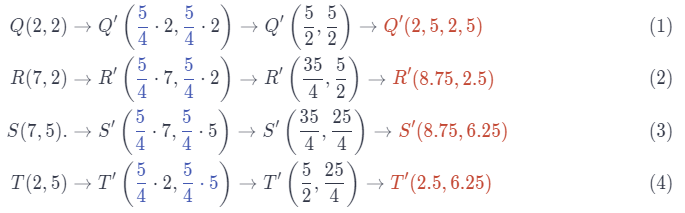e. What are the dimensions of the new room, in inches, on the blueprint? What will the dimensions of the new room be, in feet, in the new house?
The dimension of a new room on a blueprint is now:
length |QR| = |8.75 – 2.5| = 6.25 inch
height: |QT| = |6.25 – 2.5| = 3.75 inch
In feet 6.25 ∙ 4 = 25ft and 3.75 ∙ 4 = 15 ft

Question 10.
Write the algebraic representation of the dilation shown.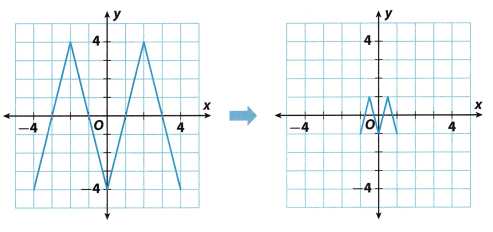Write the coordinates of some of the points on the first graph: A(-2, 4), B(2, 4), C(0, -4), and on the second graph:
A'(-$$\frac{1}{2}$$, 1) , B'($$\frac{1}{2}$$, 1) C'(0, -1). Let’s find the ratio of: the x-coordinates of corresponding points
$$\frac{-\frac{1}{2}}{-2}=\frac{\frac{1}{2}}{2}=\frac{1}{4}$$
and the ratio of the y-coordinates of corresponding points
$$\frac{1}{4}$$ = $$\frac{1}{4}$$
The scale factor is $$\frac{1}{4}$$
Algebraic rule of dilation will be (x, y) → ($$\frac{1}{4}$$x, $$\frac{1}{4}$$y)H.O.T. Focus on Higher Order Thinking

Question 11.
Critique Reasoning The set for a school play needs a replica of a historic building painted on a backdrop that is 20 feet long and 16 feet high. The actual building measures 400 feet long and 320 feet high. A stage crew member writes (x, y) → ($$\frac{1}{12}$$x, $$\frac{1}{12}$$y) to represent the dilation. Is the crew member’s calculation correct if the painted replica is to cover the entire backdrop? Explain.
The painted replica is 20 feet long and 16 feet high The actual building measures 400 feet long and 320 feet high. Lets find the ratio of length $$\frac{20}{400}$$ = $$\frac{1}{20}$$
and find the ratio of height: $$\frac{16}{320}$$ = $$\frac{1}{20}$$
So, the scale factor is $$\frac{1}{20}$$, and algebraic rule of dilation is (x, y) → ($$\frac{1}{20}$$x, $$\frac{1}{20}$$y)
A crewmember wrote wrong, the dilation is (x, y) → ($$\frac{1}{20}$$x, $$\frac{1}{20}$$y)

Algebraic Rule for Dilation Activity 8th Grade Pdf Question 12.
Communicate Mathematical Ideas Explain what each of these algebraic transformations does to a figure.
a. (x, y) → (y, -x)
In the given expression, we can notice that the x and y coordinates are replaced and that the x coordinate changed its sign.
Therefore, we can conclude that the algebraic transformation rotated a figure counterclockwise for 90°.

b. (x, y) → ( x, -y)
In the given expression, we can notice that both coordinates changed their sign Considering the fact that the positive and negative parts of both axes are spaced 180° apart, we can conclude that the algebraic transformation rotated a figure for 180°.

c. (x, y) → (x, 2y)
In the given expression, we can notice that the y coordinate is multiplied by 2 Considering the fact that the x coordinate stayed the same and the fact that the y coordinate is multiplied by a number bigger than 1, we can conclude that the given figure is vertically stretched.

d. (x, y) → ($$\frac{2}{3}$$x, y)
In the given expression, we can notice that the x coordinate is multiplied with $$\frac{2}{3}$$. Considering the fact that the y coordinate stayed the same and the fact that x coordinate is multiplied with a number smaller than 1, we can conclude that the given figure is horizontally shrunk.

e. (x, y) → (0.5x, 1.5y)